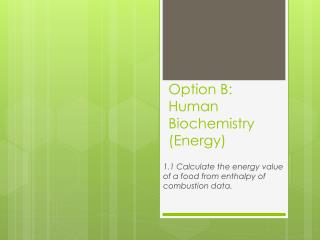DownloadDownload PresentationOption B: Human Biochemistry (Energy)

# Option B: Human Biochemistry (Energy)

Télécharger la présentation## Option B: Human Biochemistry (Energy)

- - - - - - - - - - - - - - - - - - - - - - - - - - - E N D - - - - - - - - - - - - - - - - - - - - - - - - - - -
##### Presentation Transcript

1. Option B: Human Biochemistry (Energy) 1.1 Calculate the energy value of a food from enthalpy of combustion data.

2. What is Biochemistry? • Biochemistry is the study of metabolic processes, which includes a consideration of energy changes involved

3. Why is Energy So Important? • Our cells contain biological molecules involved in complex reactions • Metabolism – sum of all these reactions • Cells get energy through respiration (starts with a simple sugar molecule like glucose) • The body needs energy-rich molecules so metabolic processes can occur • These molecules come from our diet, so it is important to know energy content of food (Joules)

4. Bomb Calorimeter • Used to measure the heat of combustion of a particular reaction • Food is placed in a container (bomb) • The bomb is placed in water • The food is ignited electrically and burned completely. • Temperature increase of water is recorded • The specific heat capacity of water (amount of energy needed to raise the temperature of 1 gram of water by 1 Kelvin), temperature change in water, and mass of water are used to calculate the heat released by the food using the following equation: q = mc Δ T

5. Diagram

6. q = mc Δ T • q = energy evolved (Joules) • m = mass of water (grams) • c = specific heat capacity of water (4.18 Jg-1K-1) • ΔT = temperature change in water (Kelvin)

7. Example 1 • A 0.78g sample of a food substance was combusted in a bomb calorimeter and raised the temperature of 105.10 g of water from 15.4°C to 30.6°C. Calculate the energy value of the food in kJ g-1.

8. Solution • Temperature rise in water = 30.6 – 15.4 = 15.2°C or 15.2 K • Specific heat capacity of water = 4.18 Jg-1K-1 • q = mc ΔT • q = (105.10 g) (4.18 Jg-1K-1) (15.2 K) • q = 6677.63 J per 0.78 g of sample heated • The energy value = = 8561.1 J g-1 or 8.56 kJ g-1 **If a question asks for an answer in J mol-1, multiply the answer in J g-1 by the molar mass ( g mol-1)

9. Example 2 • 1.50 g of glucose (C6H12O6) was completely combusted in a bomb calorimeter. The heat evolved raised the temperature of 225.00 g of water from 18.50 °C to 27.96 °C. Calculate the energy value of glucose in kJ mol-1.

10. Solution • q = mc ΔT • Temperature rise in water = 27.96 – 18.50 = 9.46°C or 9.46 K • Heat evolved = (225.00 g) (4.18 Jg-1K-1) (9.46 K) = 8897.13 J • Mr (glucose) = 180 g mol-1 • energy value of glucose = x 180 g mol-1 = 1,067,655.6 J mol-1 = 1070 kJ g mol-1

11. Example 3 • 1.00 g of sucrose,C12H22O11, was completely combusted in a food calorimeter. The heat evolved was equivalent to increasing the temperature of 631 g of water from 18.36°C to 24.58 °C. Calculate the calorific value of sucrose (in kJ mol-1) given the specific heat capacity of water in Table 2 of the Data Booklet.

12. Solution • Mrfor sucrose =342 • Heat evolved = 0.631(kg)×4.18 (kJ kg-1K-1)×6.22(K) = 16.4 kJ • Calorific value = 5.61×103 kJ mol-1

13. References • Brown, C. , & Ford, M. . (2009). Higher level chemistry developed specifically for the ibdiploma.EdinburghGate, Harlow, Essex: Pearson Education Limited.

14. Any Questions?